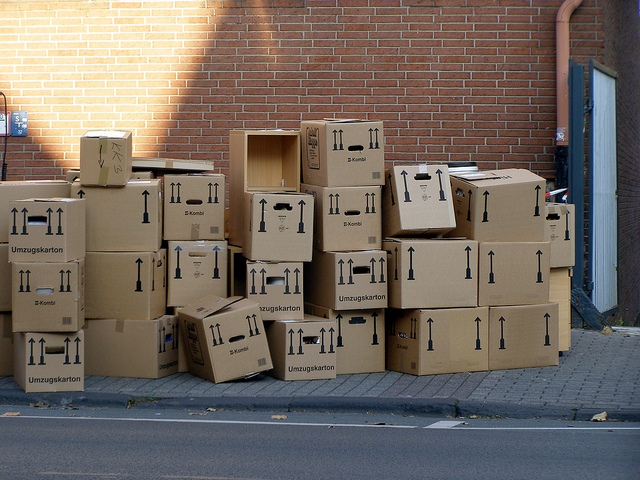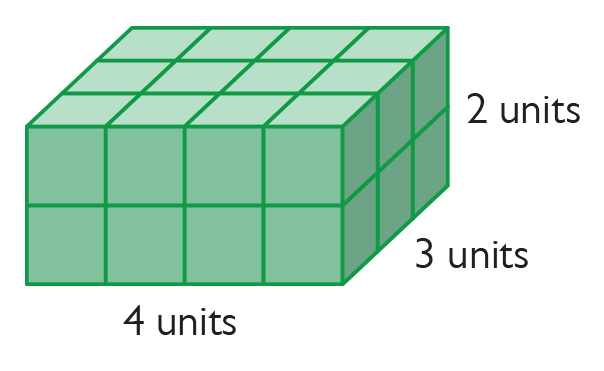# Volume Formula For Rectangular Prisms

Student View (Opens in new window)# Lesson Overview

Students build prisms with fractional side lengths by using unit-fraction cubes (i.e., cubes with side lengths that are unit fractions, such as $\frac{1}{3}$ unit or $\frac{1}{4}$ unit). Students verify that the volume formula for rectangular prisms, V = lwh or V = bh, applies to prisms with side lengths that are not whole numbers.

# Key Concepts

In fifth grade, students found volumes of prisms with whole-number dimensions by finding the number of unit cubes that fit inside the prisms. They found that the total number of unit cubes required is the number of unit cubes in one layer (which is the same as the area of the base) times the number of layers (which is the same as the height). This idea was generalized as V = lwh, where l, w, and h are the length, width, and height of the prism, or as V = Bh, where B is the area of the base of the prism and h is the height.

Unit cubes in each layer = 3 × 4

Number of layers = 5

Total number of unit cubes = 3 × 4 × 5 = 60

Volume = 60 cubic units

In this lesson, students extend this idea to prisms with fractional side lengths. They build prisms using unit-fraction cubes. The volume is the number of unit-fraction cubes in the prism times the volume of each unit-fraction cube. Students show that this result is the same as the volume found by using the formula.

For example, you can build a $\frac{4}{5}$-unit by $\frac{3}{5}$-unit by $\frac{2}{5}$-unit prism using $\frac{1}{5}$-unit cubes. This requires 4 × 3  × 2, or 24, $\frac{1}{5}$-unit cubes. Each $\frac{1}{5}$-unit cube has a volume of $\frac{1}{125}$ cubic unit, so the total volume is $\frac{24}{125}$ cubic units. This is the same volume obtained by using the formula V = lwh:$V=lwh=\frac{4}{5}×\frac{3}{5}×\frac{2}{5}=\frac{24}{125}$.$\frac{1}{5}$-unit cubes in each layer = 3 × 4

Number of layers = 2

Total number of $\frac{1}{5}$-unit cubes = 3 × 4 × 2 =  24

Volume = 24 × $\frac{1}{125}$ = $\frac{24}{125}$ cubic units

# Goals and Learning Objectives

• Verify that the volume formula for rectangular prisms, V = lwh or V = Bh, applies to prisms with side lengths that are not whole numbers.